MORE IN Analog Electronics - 1
MU Electronics and Telecom Engineering (Semester 3)
Analog Electronics - 1
December 2015
Total marks: --
Total time: --
INSTRUCTIONS
(1) Assume appropriate data and state your reasons
(2) Marks are given to the right of every question
(3) Draw neat diagrams wherever necessary

Attempt any four:
1 (a) Draw Input and Output characteristics of BJT in common emitter configuration.
5 M
1 (b) Draw small signal hybrid π equivalent circuit for npn transistor.
5 M
1 (c) Explain effect of temperature on JFET and derive equation for zero current drift.
5 M
1 (d) Calculate IB, IC & VCE for common emitter circuit.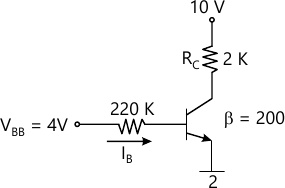5 M
1 (e) Find IB, IC & VCE for following circuit.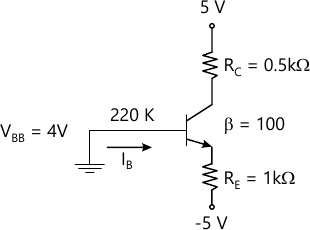5 M

2 (a) Draw output waveform for clamper and clipper circuits.

(i)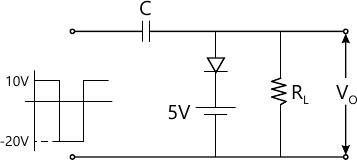(ii)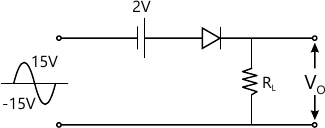10 M
2 (b) Explain construction & characteristics of n channel Enhancement MOSFET. Draw transfer characteristics & drain characteristics.
10 M

3 (a) For JFET amplifier shown below, Calculate Av, Zi, Zo.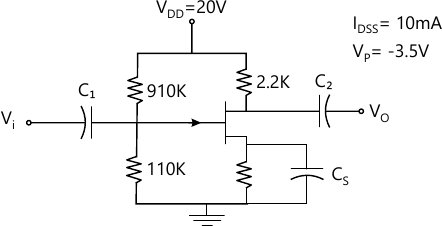10 M
3 (b) For the circuit shown below, calculate IDQ & VDSQ. It is given that ID(ON)=6 mA, VGS(ON)=8V. Vth=3V.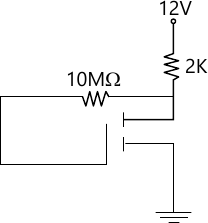10 M

4 (a) Explain the working of Wein Bridge Oscillator. Derive the expression for frequency of oscillation for sustained oscillations.
10 M
4 (b) Calculate voltage gain of FET amplifier.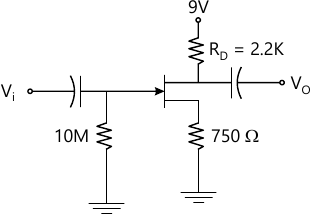Yos=40μs
IDSS=8mA
VGS off=-4V

10 M

5 (a) Draw & explain energy band diagram of MOS capacitor operating in
i) Accumulation
ii) Depletion
iii) Inversion mode
10 M
5 (b) Draw emitter follower circuit and derive an expression for voltage gain Av.
10 M

6 (a) Draw circuit diagram for phase shift oscillator & derive an expression for frequency of oscillation.
10 M
Write short notes on any two:
6 (b) (i) Photodiodes
5 M
6 (b) (ii) LC Oscillators
5 M
6 (b) (iii) Transistor as a switch
5 M
6 (b) (iv) Schottky diode
5 M

More question papers from Analog Electronics - 1